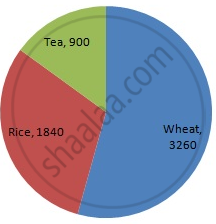Share

# Represent the Following Data with the Help of a Pie-diagram: - Mathematics

Course
ConceptCircle Graph Or Pie Chart - Drawing Pie Charts

#### Question

Represent the following data with the help of a pie-diagram:

 Items Wheat Rice Tea Production (in metric tons) 3260 1840 900

#### Solution

We know:
Central angle of a component = (component value/sum of component values x 360)
Here, total production = 6000 (in metric tons)
Thus, the central angle for each component can be calculated as follows:

 Item Production(in metric tons) Sector angle Wheat 3260 3260/6000 x 360 = 195.6 Rice 1840 1840/6000 x 360 =1 10.4 Tea 900 900/6000 x 360 = 54

Total = 6000 (in metric tons)

Now, the pie chat representing the given data can be constructed by following the steps below:
Step 1 : Draw circle of an appropriate radius.
Step 2 : Draw a vertical radius of the circle drawn in step 1.
Step 3 : Choose the largest central angle. Here, the largest central angle is 195.6o. Draw a sector with the central angle 195.6 o in such a way that one of its radii coincides with the radius drawn in step 2 and another radius is in its counter clockwise direction.
Step 4 : Construct the other sectors representing the other items in the clockwise direction  in descending order of magnitudes of their central angles.
​Step 5 : Shade the sectors with different colours and label them as shown in the figure below.​​Is there an error in this question or solution?

#### APPEARS IN

RD Sharma Solution for Mathematics for Class 8 by R D Sharma (2019-2020 Session) (2017 to Current)
Chapter 25: Data Handling-III (Pictorial Representation of Data as Pie Charts or Circle Graphs)
Ex. 25.1 | Q: 14 | Page no. 13

#### Video TutorialsVIEW ALL 

Solution Represent the Following Data with the Help of a Pie-diagram: Concept: Circle Graph Or Pie Chart - Drawing Pie Charts.
S